# MCQs on Electrical And Electronics Measurements

##### Page 8 of 19. Go to page 1 2 3 4 5 6 7 8 9 10 11 12 13 14 15 16 17 18 19
01․ A sinusoidal signal waveform, when observed on an oscilloscope, has a peak to peak amplitude of 6 cm. If the vertical sensitivity setting is 5 v/cm, then rms value of the voltage will be
15 V.
12.6 V.
11.1 V.
10.6 V.

Amplitude of the signal = 6 X 5/2 = 15 V. rms value = 15/√2 = 10.6 V.

02․ If an inductance L is connected in one arm of bridge and resistance R1, R2, R3 in other three arms
The bridge can be balanced.
The bridge can not be balanced.
The bridge is balanced for some specified value of frequency.
The bridge is balanced for some specified value of R1, R2, R3.

To balance the bridge, both magnitude and phase angle of voltages have to be balanced and this is not possible if there is only one pure inductance and three resistances because the phase of resistance is always zero and that of a pure inductance is always 90o.

03․ When testing a coil having a resistance of 10 ohms, resonance occurred when the oscillator frequency was 10 MHz and rotating capacitor was set at 500/2π pF. The effective value of the Q of the coil is
20.
25.4.
31.4.
54.2.04․ The effect of load resistance is important in measurement of
resistance of shunt.
resistance of ammeter.
resistance of shunt winding.
both A and B.

Load resistance is important when measuring low resistance. Resistance of shunt and ammeter is low.

05․ Which of following are needed both for protection and metering?
Energy meter.
Wattmeters.
Instrument transformer.
Power factor meters.

Instrument transformers are used to measure high current and voltage. They also used in the relays for protection.

06․ A voltmeter using thermocouple measures
Peak value.
rms value.
Average value.
Peak to peak value.

Since the thermocouple is based on heating effect, rms value is measured.

07․ Which of following statement are correct in case of power factor meter?
The restoring torque is provided by a controlling torque.
It consist of two coils mounted at right angles to each other.
The deflection is proportional to phase angle between field coil and crossed coil.
Both B and C.

In a power factor meter, the deflection is proportional to the phase angle between field coil and crossed coil. It consists of two coils mounted at right angles to each other.

08․ A sampling wattmeter is used to measure the average power of a load. The peak to peak voltage of a square wave is 10 V and the current is triangular wave of 5 Amp P-P as shown in the figure. The period is 20 ms. The reading in W will be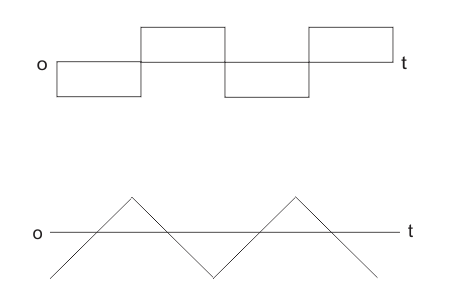0 W.
25 W.
50 W.
100 W.

Sampling wattmeter computes power from simultaneously sampled value of voltage and current. Reading of wattmeter in the circuit is given by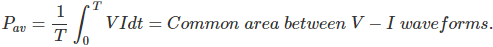In figure, total common area = 0 (Since positive and negative area are equal). So, Pav = 0.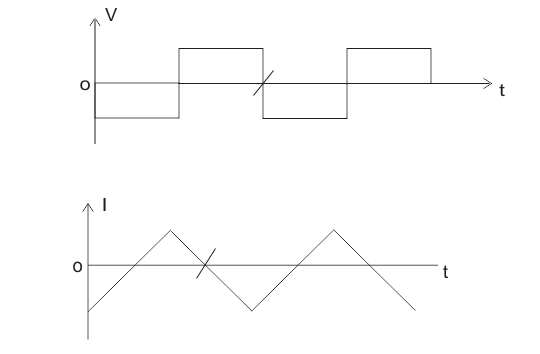09․ The time/div and voltage axes of an oscilloscope have been creased. A student connects a 1 KHz, 5 V P-P square wave calibrition pulse to channel-1 of the scope and observes the screen to be as shown in the upper trace of figure. An unknown signal is connected to channel-2 (lower trace) of the scope. If the time/div and V/div on both channels are the same, the amplitude (P-P) and period of the unknown signal are respectively
5 V, 1 ms.
5 V, 2 ms.
7.5 V, 2 ms.
10 V, 1 ms.

From the calibration pulse we can obtain voltage per division V = 5/2 = 2.5 V. Time/Division = T = 1 ms/4 = 0.25 m.sec. So amplitude (P-P) of of unknown signal is VP-P = V X 5 = 2.5 X 5 = 7.5 V. Time period T = T X 8 = 2 ms.

10․ A bridge circuit is shown in the figure below. Which one of the sentence given below is most suitable for balancing the bridge?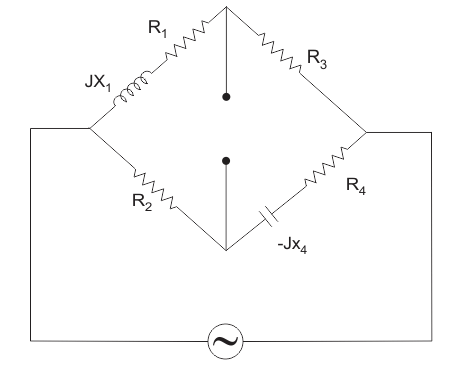To balance bridge,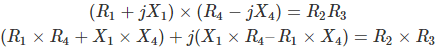Now comparing real and imaginary parts on the sides of equations,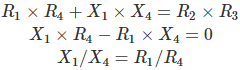From above equations it is clear that for balancing the bridge, first R4 is to be adjusted and then R1.